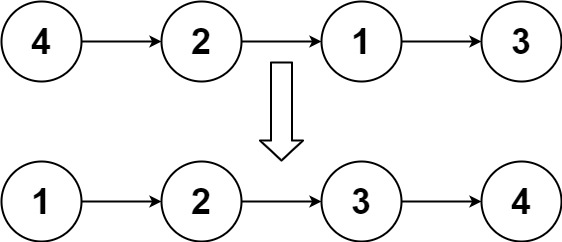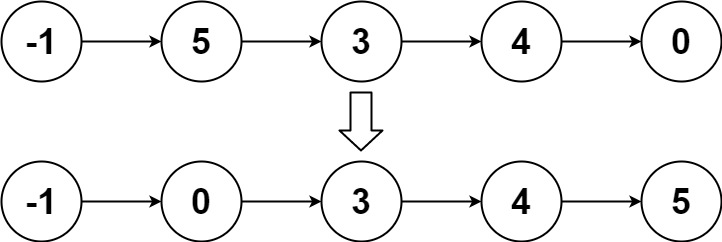# 148. Sort List | LeetCode Solution

Given the `head` of a linked list, return the list after sorting it in ascending order.

Example 1:```Input: head = [4,2,1,3]
Output: [1,2,3,4]
```

Example 2:```Input: head = [-1,5,3,4,0]
Output: [-1,0,3,4,5]
```

Example 3:

```Input: head = []
Output: []
```

Constraints:

• The number of nodes in the list is in the range `[0, 5 * 104]`.
• `-105 <= Node.val <= 105`

/**
* struct ListNode {
*     int val;
*     ListNode *next;
*     ListNode() : val(0), next(nullptr) {}
*     ListNode(int x) : val(x), next(nullptr) {}
*     ListNode(int x, ListNode *next) : val(x), next(next) {}
* };
*/
class Solution
{
public:
{

while (fast->next && fast->next->next)
{
slow = slow->next;
fast = fast->next->next;
}
return slow;
}

ListNode *merge(ListNode *h1, ListNode *h2)
{
if (h1 == NULL)
return h2;
if (h2 == NULL)
return h1;

ListNode *tail = NULL;

while (h1 != NULL && h2 != NULL)
{
if (h1->val < h2->val)
{
{
tail = h1;
}
else
{
tail->next = h1;
tail = h1;
}
h1 = h1->next;
}
else
{
{
tail = h2;
}
else
{
tail->next = h2;
tail = h2;
}
h2 = h2->next;
}
}
if (h2 != NULL)
{
tail->next = h2;
}
else if (h1 != NULL)
{
tail->next = h1;
}
}

{